# Array in Java

Challenge Inside! : Find out where you stand! Try quiz, solve problems & win rewards!
Video Tutorial## Overview

Arrays in Java are non-primitive data types that store elements of a similar data type in the memory. Arrays in Java can store both primitive and non-primitive types of data in it.

There are two types of arrays, single-dimensional arrays have only one dimension, while multi-dimensional have 2D, 3D, and nD dimensions.

## Introduction to Array in Java

In this digital world, storing information is a very crucial task to do as a programmer.

Every little piece of information needs to be saved in the memory location for future use. Instead of storing hundreds of values in different hundreds of variables, using an array, we can store all these values in just a single variable. To use that data frequently, we must assign a name, which is done by variable.

An array is a homogenous non-primitive data type used to save multiple elements (having the same data type) in a particular variable.

Arrays in Java can hold primitive data types (Integer, Character, Float, etc.) and non-primitive data types(Object). The values of primitive data types are stored in a memory location, whereas in the case of objects, it's stored in heap memory.

Assume you have to write a code that takes the marks of five students. Instead of creating five different variables, you can just create an array of lengths of five. As a result, it is so easy to store and access data from only one place, and also, it is memory efficient.

## How do Arrays in Java work?

As discussed above, an array is a data structure or container that stores the same typed elements in a sequential format.

Let's use the above example to know how arrays work in java. This is how we can implement a program to store five subject marks of a student:

But what if we store all these five variables in one single variable?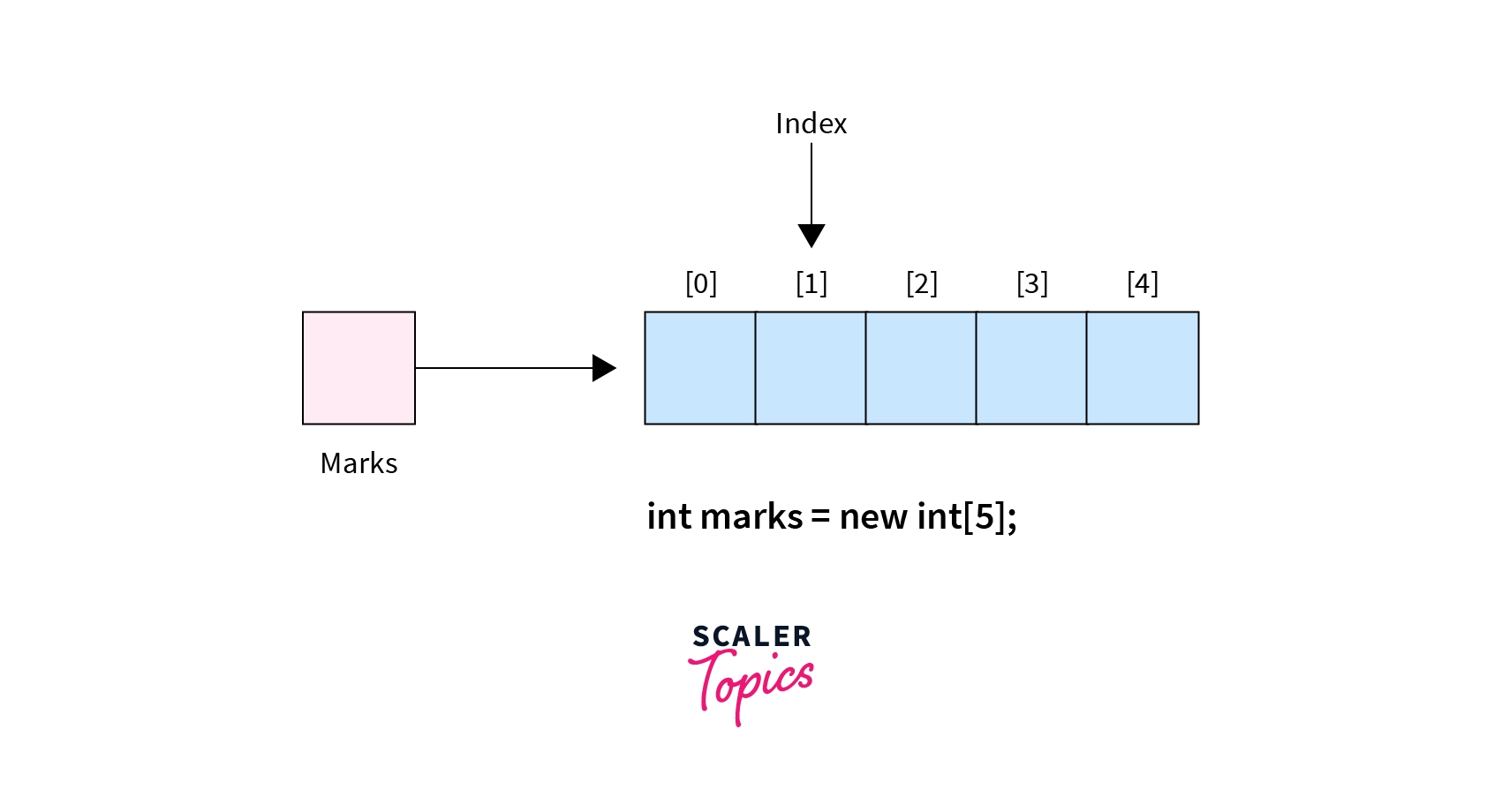All above mentioned five variables could be accessed by the index given in the square bracket, which is known as index-based variables.

The index always starts from 0 by default. So, if we have an array of 5 elements, it will have an index range from 0 to 4 by default.

In the above example, the first element is stored at index 0 and the last one at the 4th index. The below-mentioned diagram shows how the array got placed in computer memory.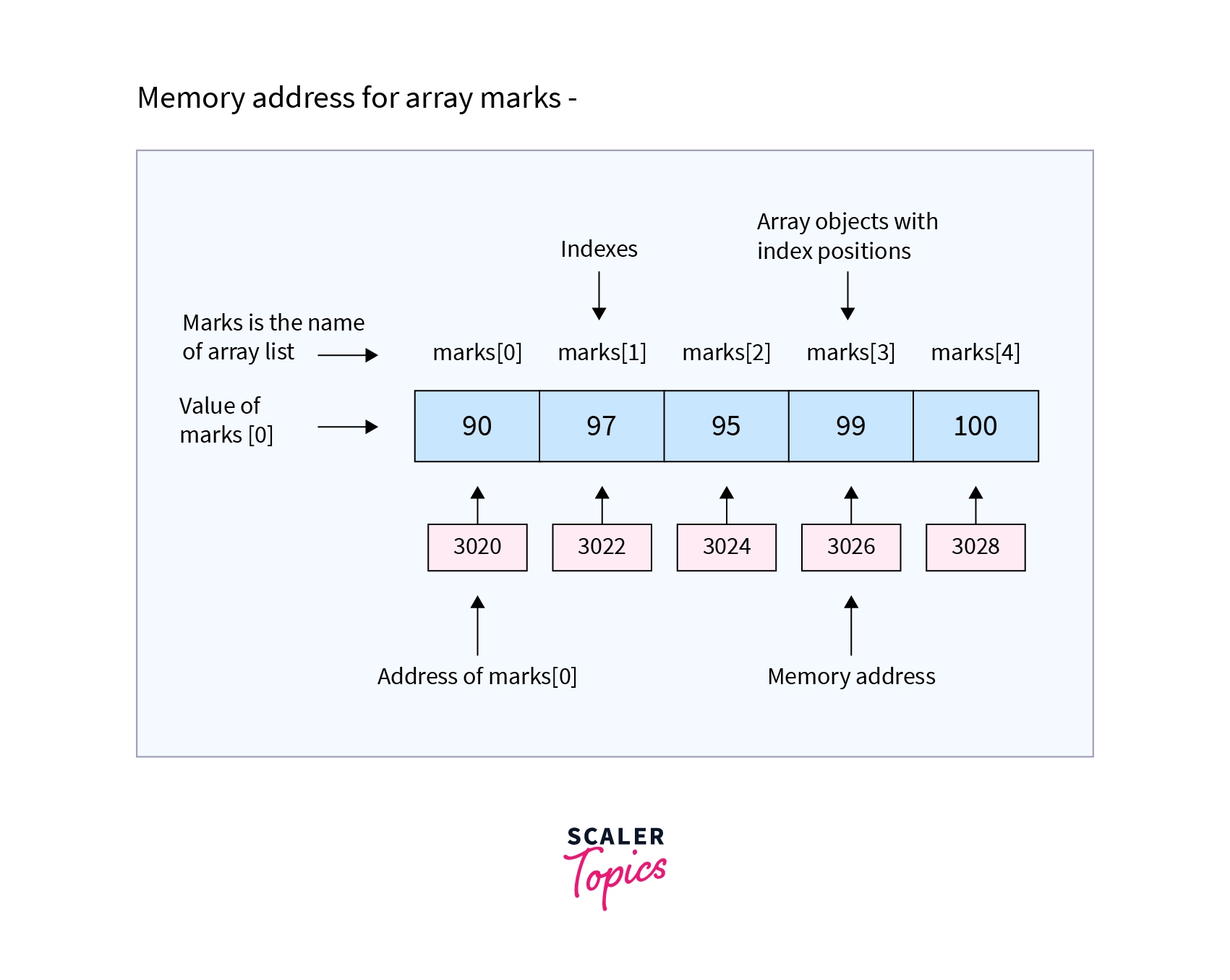## How to Create and Initialize Array in Java?

In every program, to use an array, we need to create it before it is used. We require two things to create an array in Java - data type and length.

As we know, an array is a homogenous container that can only store the same type of data. Hence, it's required to specify the data type while creating it. Moreover, we also need to put the number of elements the array will store.

Syntax:

Unlike other programming languages, while declaring an array in java, we can put [] both before and after the variable name.

The new keyword is crucial to creating an array. Without using it, we cannot make array reference variables.

Example:

### We can Initialize Arrays in Java in two Different ways -

1. Initialize using index - Once an array is created successfully, we can assign the value to any specific element using its index. Un-assigned elements automatically take the default value of the array's data type. In this case, 0 for the integer data type. On the other hand, if we try to enter more values than the size of the array, it will raise an ArrayIndexOutOfBoundsException.

Example:

1. Initialize while declaring - We can also initialize the whole array while declaring it just by using curly brackets {}. The string must be in quotes, whereas int, float, etc., do not require that.

Example:

## How to Change an Array Element?

To change a value at a specific position in Java arrays, refer to an index. By providing index value, we tell the Java compiler where we want to change the value.

For this, we need to use a Square bracket [] with an index number inside it. If somehow we entered the index number bigger than the array length, then an exception occurs of type ArrayIndexOutOfBoundsException (We will learn more about it in this article).

Example:

Let's understand clearly with a Java program.

Output:

We have created an int type array in the above code example by initializing five values. After that, we re-assigned the array element at index  from 2 to 1 just by using an array-defined index.

## Looping Through Array Elements

For performing any operation or displaying any elements, we used an index. But by using an index, we can only implement one operation at a time. If we are required to modify all elements in an array, we must write an operation for each index.

To overcome this situation, we have a looping feature in Java that follows the principle write once and execute multiple times.

There are two ways to loop through the array elements.

1. Using for Loop - In this way of looping through the array elements, we need the array's length first. This can be done by java in-built property length. If we start the for loop from 0, then the total iteration will be the array's length - 1, and if we start the for loop from 1, then the total iteration will be the length of an array.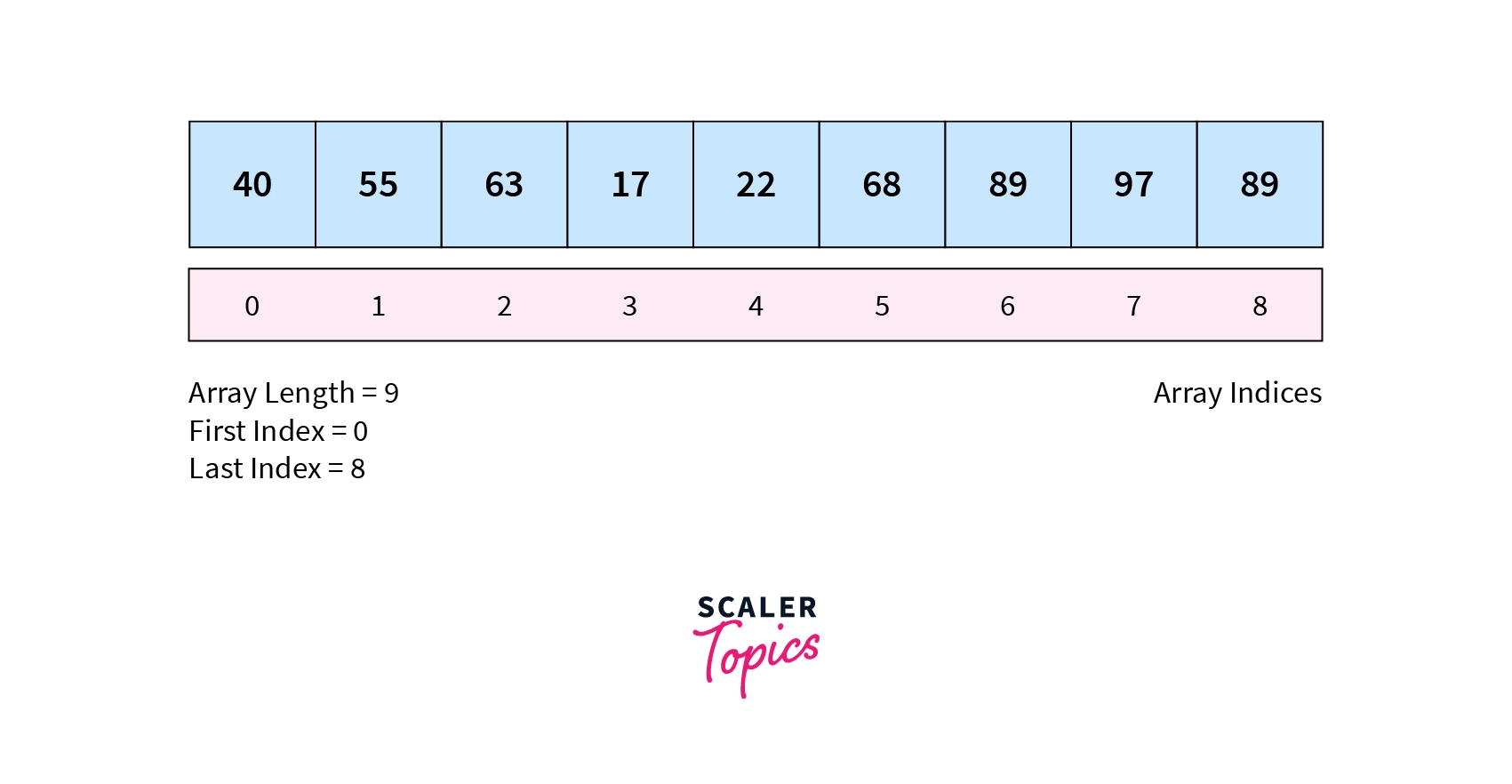As shown in the above image, there is a total of 9 value in the array, and we start looping from 0 to 8.

Example:

Output:

In the above code, we have just used a for loop to go through the array element. For the number of iterations, we find the length of the array using the array length property.

1. Using for-each loop - In this way, we use a for loop, but we do not need the length of the array. The for-each method is much easier to loop through the array element than looping for-loop. While using the for-each loop, we need to define a variable of the same type as the data type of the defined array. If not done, the compiler will throw compile time error saying- incompatible types: String cannot be converted to YourDefinedDataType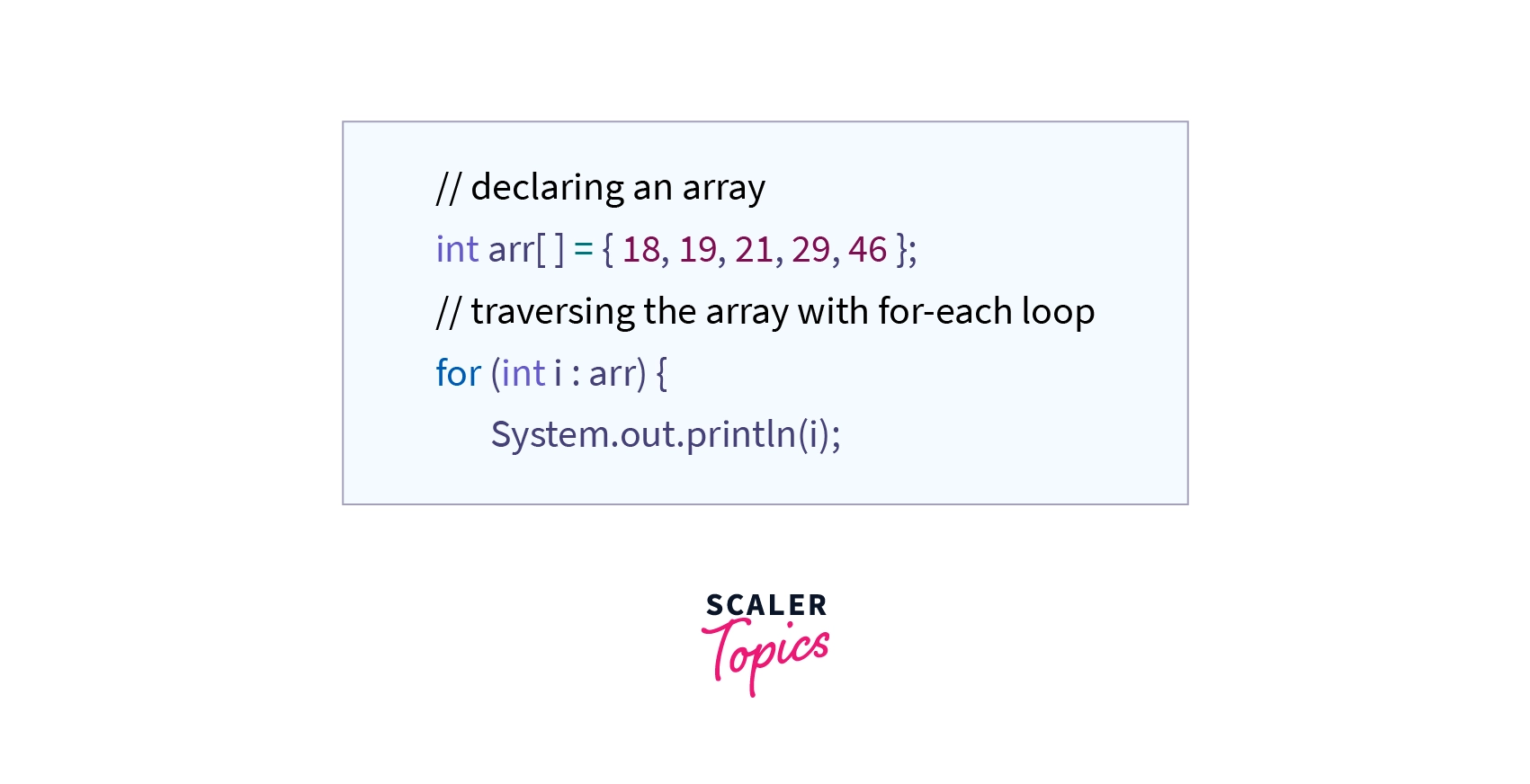Example:

Output:

The above for-each code can be read - for each String element in strArray, print the value of i.

Apart from the above two looping methods, we can also use a while loop for traversing.

## Types of Array in Java

In Java, there are two types of arrays:

### 1. Single Dimensional Array

An array that has only one subscript or one dimension is known as a single-dimensional array. It is just a list of the same data type variables.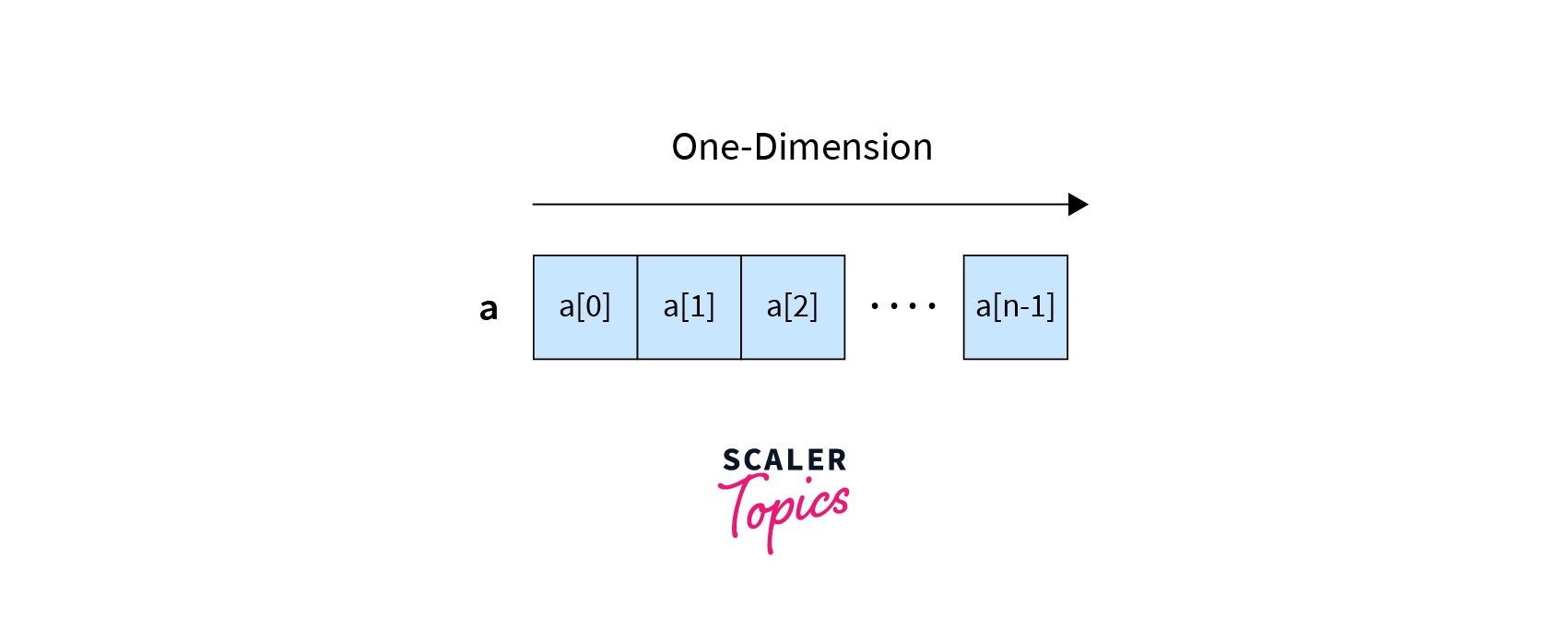One dimensional array can be of either one row and multiple columns or multiple rows and one column. For instance, a student's marks in five subjects indicate a single-dimensional array.
Example:

During the execution of the above line, JVM (Java Virtual Machine) will create five blocks at five different memory locations representing marks, marks, marks, marks, and marks.

We can also define a one-dimensional array by first declaring an array and then performing memory allocation using the new keyword.
Example:

Let's look at how we can implement a single-dimensional array in Java.

Output:

In the above example code, we created a single-dimensional array called strArray, which has only one row and multiple columns.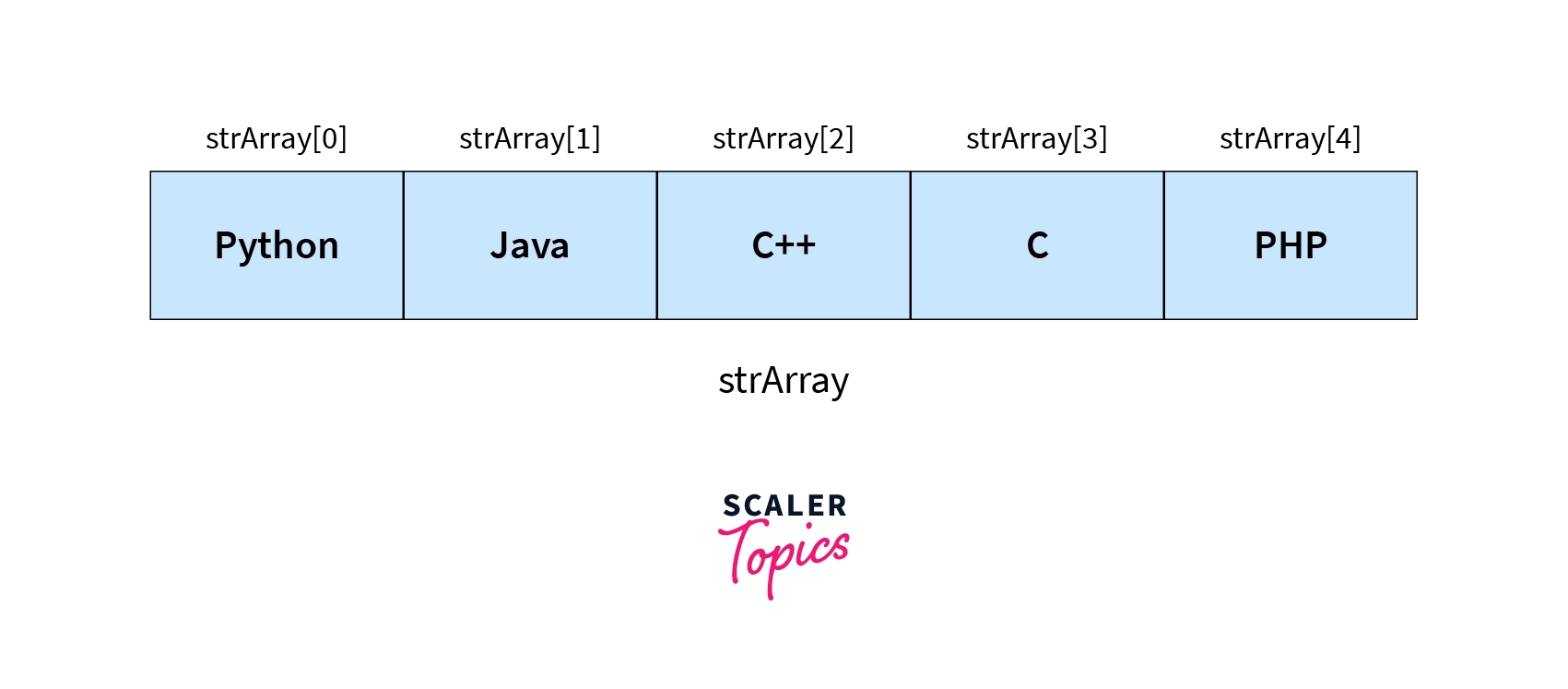### 2. Multi-Dimensional Array

In not all cases, we implement a single-dimensional array. Sometimes, we are required to create an array within an array.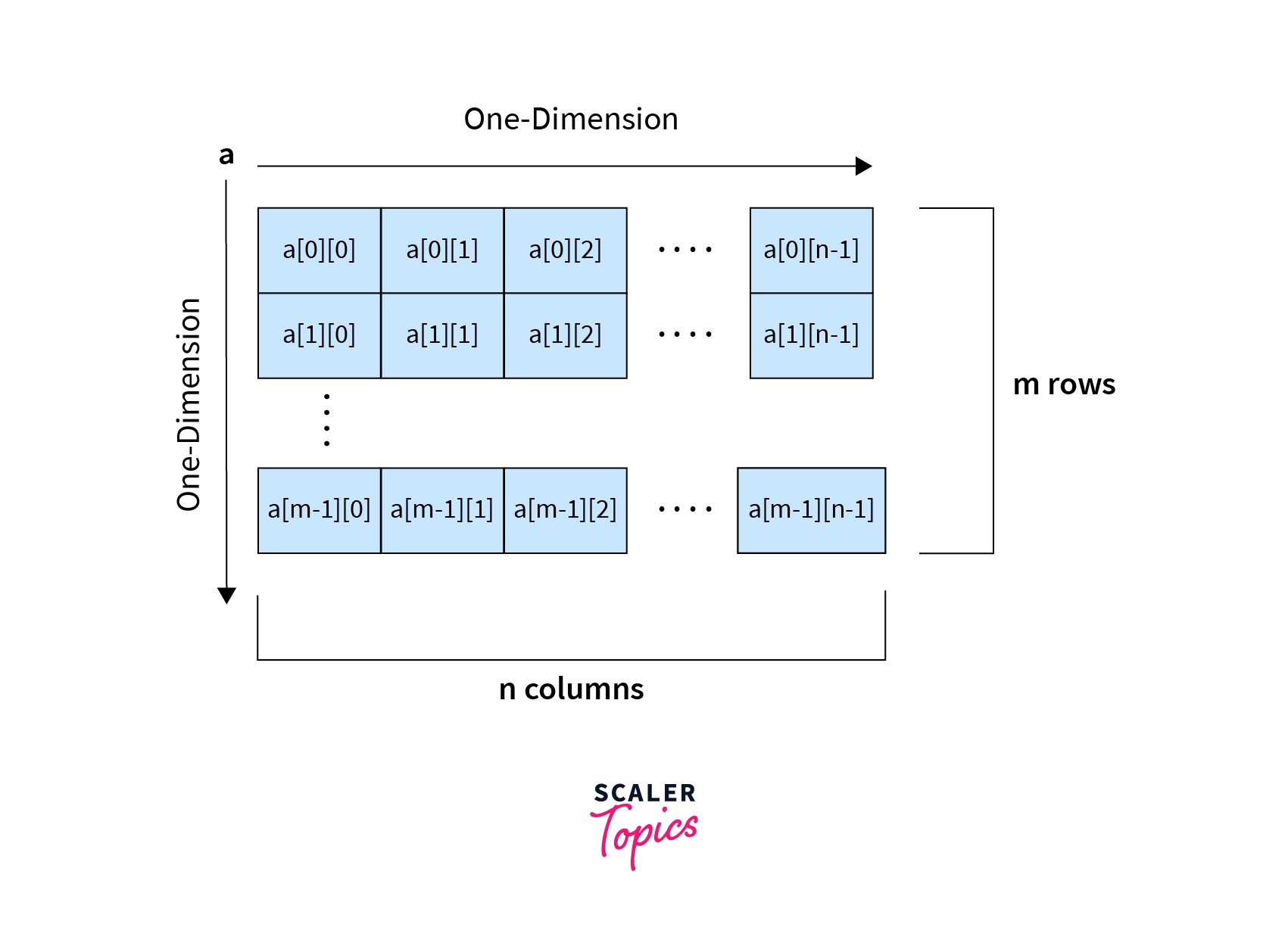Suppose we need to write a program that stores five different subjects and the marks of sixty students. In this case, we just need to implement 60 one-dimensional arrays with a length of five and then implement all these arrays in one array. Hence, we can make the 2D array with five columns and 60 rows.

A multi-dimensional array is just an array of arrays that represents multiple rows and columns. These arrays include 2D, 3D, and nD types. 2D data like tables and matrices can be represented using this type of array.

To implement a multi-dimensional array, one needs to add two square brackets instead of one.
Example:

If we want to change any specific value, we need to use two square brackets, the first representing the rows and the second representing the columns.

Now let's understand a multi-dimensional array with a famous Matrix multiplication example.

Output:

Matrix multiplication is a very famous example of a 2D array. In the above code, we declared two arrays of 3x3 dimensions. Then we created one more array with the same dimensions to store the result. Later we implemented a logic of matrix multiplication.

## Arrays of Objects

As the name suggests, an array of objects is nothing but a list of objects stored in the array. Notice that it does not store objects in an array but stores the reference variable of that object. The syntax will be the same as above.

Example:

After the above statement executes, it will create an array of objects of the Student class with a length of 3 studentObj references. For each object reference, we need to implement an object using new.

Let’s understand it more clearly with a Java program.

Output:

As you can see in the above code, we have first declared the array of objects with a length of three, which create three object reference variable obj, obj, and obj. And then initialized each object reference by using a new keyword.

## Passing Arrays to Methods

Likewise, we pass variables as a parameter and can also pass the array to methods. While passing, we do not require adding a square bracket with an array name, but we do require that in formal parameters.

Passing an array while calling methods is not required to mention the size of the array. If you do so, then it will throw the compile-time error.

Note that it is not required to use the same name of the array variable in the parameter of a function as the array name. We can use any name we want. But make sure to define the parameter variable with the same data type defined in an array in the main function, or else you will see a compilation error.

Let's understand it more clearly with a small Java program.

Output:

In the above code, we have created a static function in which we passed the array myArray from the main function. Notice that while passing an array, it is not required to use [] brackets, but in function parameters, we need to use [] brackets to define the array.

## Returning Arrays from Methods

Sometimes, we need to pass an array to a method to perform some operations and want that modified array as a return value of the function.

We already learned how we could pass arrays in methods. Let's now see how to send arrays back from the function using the return keyword.

Let's understand it more clearly with a small Java program.

Output:

Like we defined the data type of a function according to the return type in an array, we also need to define a function with an array data type. We have done the same in the above code, defined function doMultiplication() of type int[], and stored the result of this method in int type array multiplyArr.

## Anonymous Array in Java

As its name suggests, arrays having no name are called Anonymous arrays in java. This type of array is used only when we require an array instantly. We can also pass this array to any method without any reference variable.

As anonymous arrays do not have reference variables, we can not access its element, though we can use it entirely to meet our logic.

The primary reason to create an anonymous array is to implement and use an array instantly.

Syntax:

Example:

Note: In an anonymous array, we do not need to mention the size inside [] but the number of values we put inside {} becomes the size of the array.

## ArrayIndexOutOfBoundsException

Till now, we saw how to access a specific element of an array and loop through all the array elements. But what if, by mistake, we try to access an index value that is either greater than the array length or negative?

In this situation, JVM throws the runtime exception of ArrayIndexOutOfBoundsException, which points to an array accessed by an illegal index.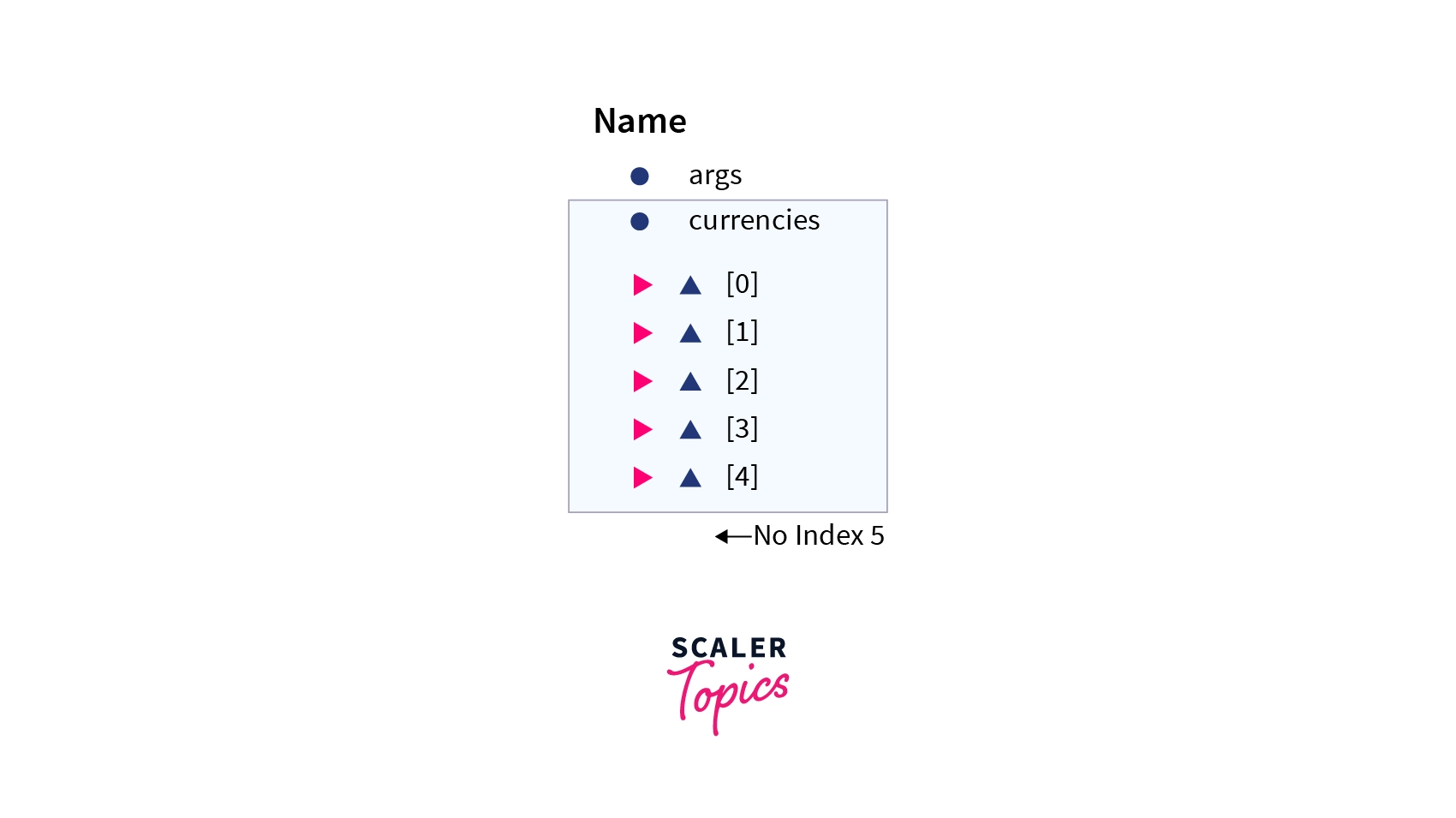If in the above example we try to access currencies then the system will give us ArrayIndexOutOfBoundsException.

Example:

Output:

In the above code, we can see that all work fine till initializing index . But, when we tried to initialize the array value at index , the compiler threw an exception, because we only have the array with a length of five.

## Cloning of Arrays in Java

Cloning an array in Java is nothing but creating a new array, where data is copied from the existing one using the clone() property.

Syntax:

Example:

Output:

When we are cloning on the multi-dimensional array, a shallow copy is performed by creating a new single array with each element referring to an original array.

Example:

Output:

As we can see, we cloned the 2D array cloneArray from myArray. Although both arrays are not the same, their elements point to the same value. Use this Java Compiler to compile your code

## Difference Between Java Array and C++ Array

Apart from the behavior of arrays, many things differ between C++ and Java talking about arrays.

• First and foremost, when we declare an array in C++, memory for the array is allocated. On the other hand, when we declare an array in Java, we only declare the reference variable, which will point to the array element once the array is initialized using a new keyword.
• Whenever we try to access an element using an index less than 0 or greater than the array length, JVM aborts the program by raising an exception of IndexOutOfBoundsException. At the same time, C++ does not have a feature to detect such errors. Hence, the program goes into an infinity loop until the max buffer length is not exceeded.

• As far as an array of objects is concerned, C++ will likely implement the constructed array of objects in a single operation. On the other hand, Java needs multiple steps to perform an array of objects.

• Like arrays in Java, C++ does not have a length property to calculate the array length every time we want. We need to store the length of an array in the C++ program.

• We can access an array element value randomly just by using the index indicated by the array.
• At a time, we can store lots of values in arrays.
• It becomes easier to create and work with the multi-dimensional arrays.

• Arrays in Java do not have in-built remove or add methods.
• In Java arrays, we need to specify the size of the array. So there might be a change of memory wastage. So, it is recommended to use ArrayList.
• Arrays in Java are strongly typed.

## Conclusion

• Array in Java is a non-primitive data type used to store multiple values of the same data type.
• Elements of the array can be accessed by the index ranging from 0 to array length - 1.
• We can use for loop and for-each loop for looping through all the array elements.
• There are two arrays in Java: Single and Multi-dimensional, where single has one dimension, and multi includes 2D, 3D, and nD dimensions.
• Likewise primitive data types, we can also implement an array of objects in Java.
• We also learned to pass the array in methods and return the array from the methods.
• Array with no name is called an anonymous array performed for instant use.
• We discussed duplicating arrays using the clone() property for both single and multi-dimensional arrays.

Q. What is an Array?

A. An array is a homogenous non-primitive data type used to save multiple same-type data values in a particular variable.

Q. Are arrays reference types in Java?

A. Yes. In Java, an array is a container of objects treated as references.

Q. Are arrays primitive data types in Java?

A. No. Arrays in Java are non-primitive data types. But, an array can hold both primitive and non-primitive data type variables.

Q. an you increase the size of an array in Java?

A. No. As we discussed earlier, we need to specify its size in Java first. Once we declare the array, we can not change or update its size runtime.